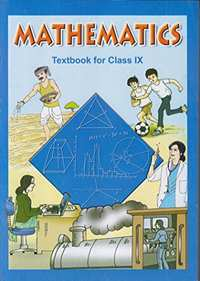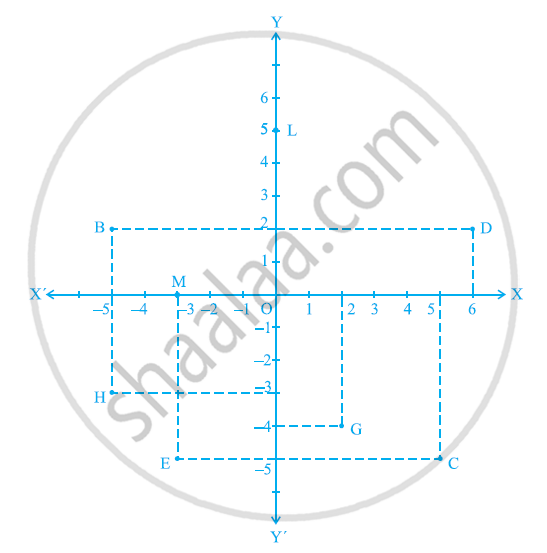Share

Books Shortlist

NCERT solutions for Class 9 Mathematics chapter 3 - Coordinate Geometry

Mathematics Textbook for Class 9

NCERT Mathematics Class 9Chapter 3: Coordinate Geometry

Ex. 3.10Ex. 3.20Ex. 3.30

Chapter 3: Coordinate Geometry Exercise 3.10 solutions [Page 53]

Ex. 3.10 | Q 1 | Page 53

How will you describe the position of a table lamp on your study table to another person?

Ex. 3.10 | Q 2 | Page 53

(Street Plan) : A city has two main roads which cross each other at the centre of the city. These two roads are along the North-South direction and East-West direction.

All the other streets of the city run parallel to these roads and are 200 m apart. There are 5 streets in each direction. Using 1cm = 200 m, draw a model of the city on your notebook. Represent the roads/streets by single lines.

There are many cross- streets in your model. A particular cross-street is made by two streets, one running in the North - South direction and another in the East - West direction. Each cross street is referred to in the following manner : If the 2nd street running in the North - South direction and 5th in the East - West direction meet at some crossing, then we will call this cross-street (2, 5). Using this convention, find:-

(i) how many cross - streets can be referred to as (4, 3).

(ii) how many cross - streets can be referred to as (3, 4).

Chapter 3: Coordinate Geometry Exercise 3.20 solutions [Page 60]

Ex. 3.20 | Q 1.1 | Page 60

Write the answer of the following question:-

What is the name of horizontal and the vertical lines drawn to determine the position of any point in the Cartesian plane?

Ex. 3.20 | Q 1.2 | Page 60

Write the answer of each of the following questions:-

What is the name of each part of the plane formed by these two lines?

Ex. 3.20 | Q 1.3 | Page 60

Write the answer of each of the following questions:-

Write the name of the point where these two lines intersect.

Ex. 3.20 | Q 2 | Page 60

See the given figure, and write the following:-

(i) The coordinates of B.

(ii) The coordinates of C.

(iii) The point identified by the coordinates (–3, –5).

(iv) The point identified by the coordinates (2, – 4).

(v) The abscissa of the point D.

(vi) The ordinate of the point H.

(vii) The coordinates of the point L.

(viii) The coordinates of the point M.Chapter 3: Coordinate Geometry Exercise 3.30 solutions [Page 65]

Ex. 3.30 | Q 1 | Page 65

In which quadrant or on which axis do each of the points (– 2, 4), (3, – 1), (– 1, 0), (1, 2) and (– 3, – 5) lie? Verify your answer by locating them on the Cartesian plane.

Ex. 3.30 | Q 2 | Page 65

Plot the points (x, y) given in the following table on the plane, choosing suitable units of distance on the axes.

 x -2 -1 0 1 3 y 8 7 -1.25 3 -1

Chapter 3: Coordinate Geometry

Ex. 3.10Ex. 3.20Ex. 3.30

NCERT Mathematics Class 9NCERT solutions for Class 9 Mathematics chapter 3 - Coordinate Geometry

NCERT solutions for Class 9 Maths chapter 3 (Coordinate Geometry) include all questions with solution and detail explanation. This will clear students doubts about any question and improve application skills while preparing for board exams. The detailed, step-by-step solutions will help you understand the concepts better and clear your confusions, if any. Shaalaa.com has the CBSE Mathematics Textbook for Class 9 solutions in a manner that help students grasp basic concepts better and faster.

Further, we at shaalaa.com are providing such solutions so that students can prepare for written exams. NCERT textbook solutions can be a core help for self-study and acts as a perfect self-help guidance for students.

Concepts covered in Class 9 Mathematics chapter 3 Coordinate Geometry are Introduction of Coordinate Geometry, Cartesian System, Plotting a Point in the Plane If Its Coordinates Are Given.

Using NCERT Class 9 solutions Coordinate Geometry exercise by students are an easy way to prepare for the exams, as they involve solutions arranged chapter-wise also page wise. The questions involved in NCERT Solutions are important questions that can be asked in the final exam. Maximum students of CBSE Class 9 prefer NCERT Textbook Solutions to score more in exam.

Get the free view of chapter 3 Coordinate Geometry Class 9 extra questions for Maths and can use shaalaa.com to keep it handy for your exam preparation

S